Next: Periodic Signals Up: Sinusoids, amplitude and frequency Previous: Synthesizing a sinusoid   Contents   Index

# Superposing Signals

If a signal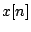has a peak or RMS amplitude(in some fixed window), then the scaled signal(where) has amplitude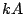. The mean power of the scaled signal changes by a factor of. The situation gets more complicated when two different signals are added together; just knowing the amplitudes of the two does not suffice to know the amplitude of the sum. The two amplitude measures do at least obey triangle inequalities; for any two signalsand,If we fix a window fromto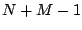as usual, we can write out the mean power of the sum of two signals:where we have introduced the covariance of two signals:The covariance may be positive, zero, or negative. Over a sufficiently large window, the covariance of two sinusoids with different frequencies is negligible compared to the mean power. Two signals which have no covariance are called uncorrelated (the correlation is the covariance normalized to lie between -1 and 1). In general, for two uncorrelated signals, the power of the sum is the sum of the powers: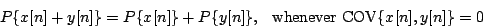Put in terms of amplitude, this becomes:This is the familiar Pythagorean relation. So uncorrelated signals can be thought of as vectors at right angles to each other; positively correlated ones as having an acute angle between them, and negatively correlated as having an obtuse angle between them.

For example, if two uncorrelated signals both have RMS amplitude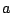, the sum will have RMS amplitude. On the other hand if the two signals happen to be equal--the most correlated possible--the sum will have amplitude, which is the maximum allowed by the triangle inequality.Next: Periodic Signals Up: Sinusoids, amplitude and frequency Previous: Synthesizing a sinusoid   Contents   Index
Miller Puckette 2006-12-30